Homework Help Question & Answers

# A Carnot engine whose hot-reservoir temperature is 400 ∘C has a thermal efficiency of 45 %...

A Carnot engine whose hot-reservoir temperature is 400 ∘C has a thermal efficiency of 45 % . By how many degrees should the temperature of the cold reservoir be decreased to raise the engine's efficiency to 58 % ?

#### Homework Answers

Answer #1

Efficiency of carnot engine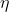= 1 - ( Tc / Th )

hot-reservoir temperature Th =  400 ∘C

0.45 = 1 - [ Tc / (400+2730) ]

Tc = 370.15 K ----------------------(1)

Now to get the efficiency 58%

0.58 = 1 - [ Tc / (400+273) ]

Tc = 282.66 K -------------------(2)

So from (1) and (2) it clear that

temperature should be decreased by

370.15 - 282.66 = 87.49 ∘C

Good Luck !

Know the answer?
Your Answer:

#### Post as a guest

Your Name:

What's your source?

#### Earn Coin

Coins can be redeemed for fabulous gifts.

Not the answer you're looking for? Ask your own homework help question. Our experts will answer your question WITHIN MINUTES for Free.
Similar Homework Help Questions
• ### A Carnot engine has an efficiency of 0.45. The Kelvin temperature of its hot reservoir is...

A Carnot engine has an efficiency of 0.45. The Kelvin temperature of its hot reservoir is quadrupled, and the Kelvin temperature of its cold reservoir is doubled. What is the efficiency that results from these changes?

• ### A Carnot engine has an efficiency of 45 % and takes in heat from a high-temperature...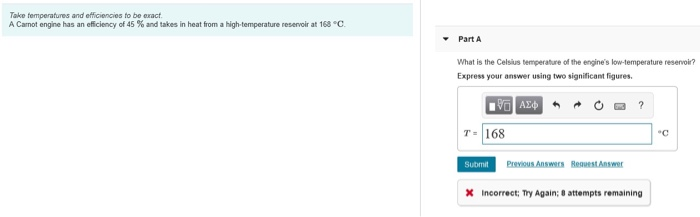A Carnot engine has an efficiency of 45 % and takes in heat from a high-temperature reservoir at 168 ∘C. What is the Celsius temperature of the engine's low-temperature reservoir? T = _____ °C (Answer is NOT 168°C) Take temperatures and efficiencies to be exact. A Carnot engine has an efficiency of 45 % and takes in heat from a Nigh-temperature reservoir at 168 °C Part A What is the Celsius temperature of the engine's low-temperature reservoir? Express your answer...

• ### A Carnot engine has an efficiency of 0.445, and the temperature of its cold reservoir is...

A Carnot engine has an efficiency of 0.445, and the temperature of its cold reservoir is 434 K. (a) Determine the temperature of its hot reservoir. (b) If 5610 J of heat is rejected to the cold reservoir, what amount of heat is put into the engine?

• ### A Carnot engine operates with an efficiency of 29% when the temperature of its cold reservoir...

A Carnot engine operates with an efficiency of 29% when the temperature of its cold reservoir is 295 K. Assuming that the temperature of the hot reservoir remains the same, what must be the temperature of the cold reservoir in order to increase the efficiency to 33%?

• ### Chapter 20, Problem 023 A Carnot engine whose low-temperature reservoir is at 11.4°C has an efficiency...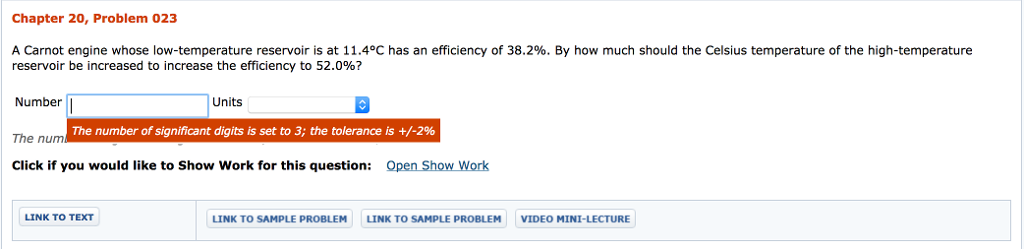Chapter 20, Problem 023 A Carnot engine whose low-temperature reservoir is at 11.4°C has an efficiency of 38.2%. By how much should the Celsius temperature of the high-temperature reservoir be increased to increase the efficiency to 52.0%? Number The nunm Click if you would like to Show Work for this question: Open Show Work Units The number of significant digits is set to 3; the tolerance is +/-2% LINK TO TEXT LINK TO SAMPLE PROBLEM LINK TO SAMPLE PROBLEM VIDEO...

• ### A Carnot engine operates between temperatures of 710 and 339 K. To improve the efficiency of...

A Carnot engine operates between temperatures of 710 and 339 K. To improve the efficiency of the engine, it is decided either to raise the temperature of the hot reservoir by 41 K or to lower the temperature of the cold reservoir by 41 K. Which change gives the greatest improvement? raise the temperature of the hot reservoir? or lower the temperature of the cold reservoir? Justify your answer by calculating the efficiency in each case. raise TH ____ lower...

• ### An engine does 20 J of work and exhausts 15 J of waste heat during each cycle. What is the engine's thermal efficiency...

An engine does 20 J of work and exhausts 15 J of waste heat during each cycle. What is the engine's thermal efficiency? If the cold-reservoir temperature is 30 degrees C, what is the minimum possible temperature in degrees C of the hot reservoir?

• ### A Carnot engine has an efficiency of 26.7%. It operates between constant-temperature reservoirs differing in temperature...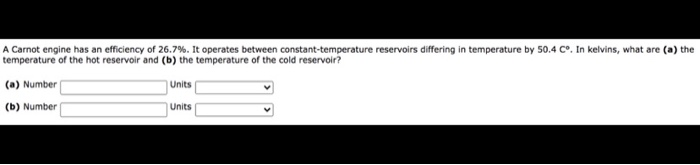A Carnot engine has an efficiency of 26.7%. It operates between constant-temperature reservoirs differing in temperature by 50.4 Cº. In kelvins, what are (a) the temperature of the hot reservoir and (b) the temperature of the cold reservoir? (a) Number Units (b) Number Units

• ### Chapter 20, Problem 023 X Incorrect. A Carnot engine whose low-temperature reservoir is at 16.7°C has...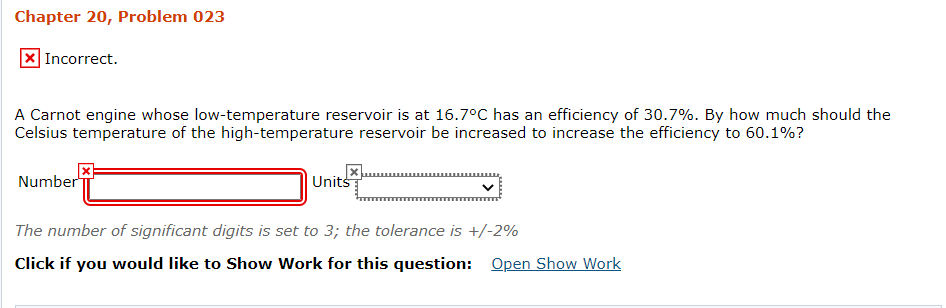Chapter 20, Problem 023 X Incorrect. A Carnot engine whose low-temperature reservoir is at 16.7°C has an efficiency of 30.7%. By how much should the Celsius temperature of the high-temperature reservoir be increased to increase the efficiency to 60.1%? Number Units The number of significant digits is set to 3; the tolerance is +/-2% Click if you would like to Show Work for this question: Open Show Work

• ### i need correct solution A Carnot engine has an efficiency of 31.2%. It operates between constant-temperature...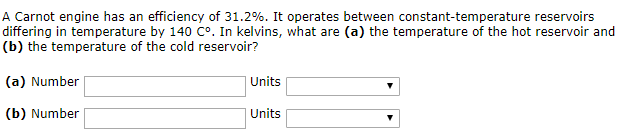i need correct solution A Carnot engine has an efficiency of 31.2%. It operates between constant-temperature reservoirs differing in temperature by 140 C°. In kelvins, what are (a) the temperature of the hot reservoir and (b) the temperature of the cold reservoir? (a) Number Units Units (b) Number

Free Homework App

Scan Your Homework
to Get Instant Free Answers
Need Online Homework Help?

Get Answers For Free
Most questions answered within 3 hours.